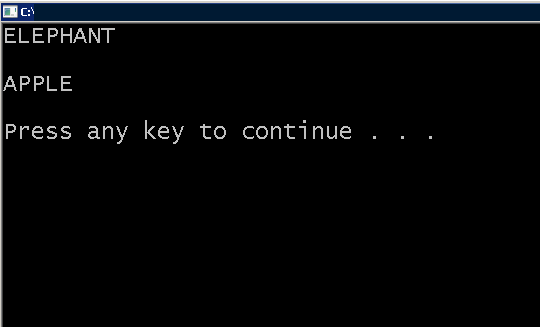## C Programming Tutorial – Strings and Pointers

In the previous lesson, you learned about C string and how they are important to manipulate text in a C program. In this article, you will learn how strings can be accessed and used with pointers.

Learn C programming basics and pointer concepts before you begin with this lesson. If you are familiar with them, skip and continue reading the next section.

Pointer is a special variable that can store the value of string constant, just like character array. But, there are some advantages of using pointers.

Let us see the initialization of string constant.

``````char manager = "David";
char *p = "Harry";``````

Both methods is simple and straightforward, but you cannot assign another string to a character array. It is only possible with pointers.

For example,

``````char manager = "Bruce";
char supervisor;
char *p = "Mary";
char *s;

supervisor = manager; /* will give error */

*s = *p;                      /* No error */

*s = "Tang";               /* is valid */

supervisor= "George";  /* Not valid */``````

## Accessing Characters in a String

The most popular method to access individual characters from a string is treating the string like an array and loop through each character.

For example,

``````int i = 0;
while(name[i] != 'int i = 0;
while(name[i] != '\0'){
printf("%c", name[i]);
}
getch();
return 0;
}'){
printf("%c", name[i]);
}
getch();
return 0;
}``````

You can do the same with pointers. Consider the pointer version of above.

``````char *p = "elephant";
int i;

while (*p!= 'char *p = "elephant";
int i;
while (*p!= '\0')
{
printf("%c",*p);
p++;
}')
{
printf("%c",*p);

p++;

}``````

The output of both is going to be same.

## String and Pointer Example Program

The following program does all the string manipulation we discussed in the article.

``````#include <stdio.h>

#include <stdlib.h>

int main()
{

char fruit = "APPLE";

char *p = "ELEPHANT";

char *s;

int i;

s = p;

while (*s!= '#include <stdio.h>
#include <stdlib.h>
int main()
{
char fruit = "APPLE";
char *p = "ELEPHANT";
char *s;
int i;
s = p;
while (*s!= '\0')
{
printf("%c",*s);
s++;
}
printf("\n\n");
/* Standard Array Technique */
i = 0;
while( fruit[i] != '\0')
{
printf("%c", fruit[i]);
i++;
}
printf("\n\n");
system("PAUSE");
return 0;
}')
{

printf("%c",*s);

s++;

}

printf("\n\n");

/* Standard Array Technique */

i = 0;

while( fruit[i] != '#include <stdio.h>
#include <stdlib.h>
int main()
{
char fruit = "APPLE";
char *p = "ELEPHANT";
char *s;
int i;
s = p;
while (*s!= '\0')
{
printf("%c",*s);
s++;
}
printf("\n\n");
/* Standard Array Technique */
i = 0;
while( fruit[i] != '\0')
{
printf("%c", fruit[i]);
i++;
}
printf("\n\n");
system("PAUSE");
return 0;
}')
{
printf("%c", fruit[i]);

i++;

}

printf("\n\n");

system("PAUSE");

return 0;

}``````

## OutputOutput – String and Pointer

Related Articles:-